A Statistical Approach to Parsing of Czech

Daniel Zeman

ÚFAL, MFF, Univerzita Karlova, Praha

Thise present paper is based on my dissertation that was defended at Charles University in May 1997. I introduce here a simple probabilistic model of Czech syntax, based on training data extracted from a text corpus. Then I try to employ this model in building syntaxctic trees of Czech sentences. In this paper, a brief description of the model is given, as well as a summary of the test results. The work is one of the first attempts to use the statistical learning methods for parsing of Czech. It is to be said here that the results procedures used to parse of parsing of English cannot be easily ported to Czech, since because some specific characteristics of Czech (free word order, and wide-ranging rich flexion implying a huge number of word forms) cause a different behaviour of a parser. The work made presented is neither final, nor satisfying solution of the problem. It rather presents demonstrates the primary results and proposes some basic ideas of further research.

1. Introduction
2. The task of parsing text (or speech) is something that a human being solves many times every day. Reading a text or listening to someone, you one needs to parse each sentence if you s/he wants to understand the utterance. You One needs to know, for instance, that in the sentence ”The child ate the yellow apple.” the word ”yellow” forms a noun phrase combines with the word ”apple” rather than with ”child”. Wrongly parsed sentences would have a significantly different meanings.

Although a native speaker is not probably not aware of that, s/he actually is parsing constantly. A fForeigners may have some difficulties if his their mother tongues has have an essentially different syntax. He They may be parsing knowingly. And if you we want the sentence meanings to be distinguished by a computer, e.g. when doing a in machine translation, you will we have to deal with syntax more than you even would like to.

On the opposite other side, when writing a text, a human has to know about the syntax in order to make form grammatically correct sentences. Particularly in Czech, where many syntactic relations are expressed by choosing adequate word forms, there are many opportunities to make a ”syntax error”. Today we are used to write texts in a word processor and to have we expect our errors to be corrected by the computer. Spell Spell-checkers find for us any non-existing word forms we write down. But even a spell spell-checker will fail to recognise a syntactically wrong sentence formed by word forms that are allowable acceptable in another context. Instead, we need a grammar checker which is another important application to employ at least some steps of a syntax syntactic analyser.

What do we actually mean by ”parsing”? In general, it means finding the grammatical structure of a sentence. There might be several ideas what does this structure looks like; however, we are not going to consider the various possible approaches, rather we will present the one we will be dealing with. At this point, let us say that in our approach the structure of a sentence is represented by a dependency tree where the nodes are the words from the sentence, and the edges are dependencies between them. (Some A more formal definition of the structure will be given later.) So, we can say that the task of parsing involves constructing a dependency tree, given a ”plain”string of word forms, constituting a sentence.

With statistical modelling, we will look at parsing as a random process, and we will try to predict its behaviour. We will have at our disposal a sample of previous the output of the process, which represents an incomplete knowledge about the process. Our goal will be to analyse this sample statistically and to construct a model — a probabilistic distribution allowing us to say, which dependency tree is the most probable for a given plain sentence.

In Section 2 we give a formal definition of the parsing problem. The Section 3 where wWe define the model in Section 3, which is a theoretical background; then, in Section 4 we describe the algorithm used to build the model and to employ it in parsing. In Section 5 we introduce methods of testing the model, and we present the results of the experiments. Finally, in Section 6, we summarise the results and discuss several ideas about improvements and directions of further research.

3. Sentences and Dependency Trees
4. A plain sentence, or simply a sentence, is a sequence of words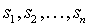. Generally we do not need not to know what is a word. It can be simply the word form which appeared in the sentence but it might also contain some morphological information, the lemma and so on. Additional symbols such as numbers and interpunction are considered separate words.

A dependency tree is formed by a set of nodes V and a set of edges H. Each node corresponds to a word from the sentence, except of for the root of the tree. Each edge represents a dependency between a superior governor and its subordinate constituents. The definition of a tree requires that there is exactly one node superior to any constituent of the sentence. However, every node may have any number of subordinate constituents. The root of the tree is identified by a special node #. It does not correspond to any word from the sentence, it only serves as the root markingmarker. Each tree has exactly one # node. The nodes are ordered so that we can define depth-first and width-first tree searches.

Note that we have not defined any rule saying how a tree is constructed given a sentence. Rather we are hoping that our statistical model discovers those rules and describes them in terms of probability. So our intuitive knowledge of how a tree is constructed will be used only indirectly, as we will define the model.

5. The Statistical Model
6.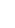Let S be a sentence of n words. According to our definition, the dependency tree of that sentence will consist of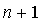nodes (including the root #) and n edges. There are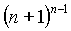such trees. Among them, we want to select the most probable one, i.e. the tree that a human would assign to the given sentence most often. Formally, we are looking for a tree M, defined as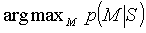From the Bayes’ rule we get:Here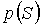is only a constant that has no influence on the result: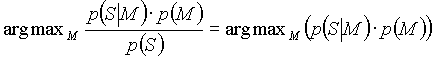The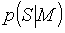is the probability that the tree M was constructed just exactly from the sentence S. Since we are able to get back the original sentence from the tree (e.g. by having preserved the original word forms and original word order as an additional info to each node), we understand theto be only a technical tool. It is a binary function giving one if the tree is correct and corresponding to S, and zero otherwise.

The real strength then resides in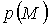, the probability of the tree. Unfortunately we are not able to model it directly: since there is such a huge big number of trees, we cannot get a parsing sample large enough to estimate their probabilities. We are forced to simplify the task essentially and to approximate the probabilities in some way, which on the other hand cannot be done without a loss of accuracy.

First, we reduceto the probability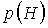of an edge sequence H. We can do that because every tree is well-defined by the set of its set of edges. There is a small problem with the fact that not every sequence of edges on a given set of nodes makes is a tree. Nonetheless, we can define a special treethat does not correspond to any real sentence and assign it to all wrong edge sequences. We can think of this tree as of an empty tree, containing only the root. It is clear that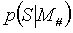will be always zero. You We see that if we replace the probability of the tree is replaced by with the probability of an edge sequence, the most probable tree will be just. But However, the proportions among the other trees are left intact, so that we have not distorted the results so far.

We can breakdown as follows: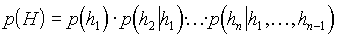Now it is the time to reduce the complexity and the number of parameters of our model. We cannot expect that our sample (training) data will give us ability make it possible to us to estimate probabilities that are conditional in more than one dimensions. The longer vectors of edges we bear in mindconsider, the lower is the recall (the less fewer times we find a vector in the training sample). On the other hand, if we reduce the vectors to one member, getting unconditional probabilities, we lose significantly on the precision. Let us have a look at the unconditional, so-called unigram language model. We suppose that for every edgeit holds thatThen we see that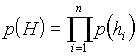Now the problem is much more simple because we can estimate the edge probabilitiesby the relative frequencies from the training data,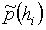. Because of our suspicion Since we suspect that the unigram model is insufficiently precise, we will also study the bigram model where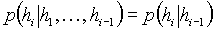and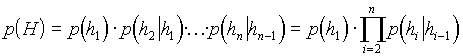If we have a large sample of training data, we shall be able to estimate probabilities of pairs of edges, too. However, we face here an additional problem, the one of specifying the order of the edges. If we want to make the probability conditional on the preceding edge, we have to specify which edge is preceding to the other precedes the given one. That This is why we have required that the nodes of the dependency tree are ordered and that we can define depth-first or width-first search. Now we can assign the same order to the edges since each node (except of the root) has exactly one input edge. We do not know whether there is a stronger correlation between depth-first neighbours, or between the width-first ones, and whether there is any correlation at all. So we are going to try both orders in our experiments.

Finally we should want to emphasise one aspect of the above language model definition. We transposed the probability of a tree to the probability of an edge sequence, where the word sequence implies that a predefined edge order exists. It means that among two edge permutations, at most one may be considered correct, while the other is assigned the special tree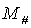.

7. The Algorithms
8. The procedure will consist of two phases, one of training and another of parsing. During the training period we will step go through the training data, read dependency trees and count frequencies of all the edges or edge pairs, respectively. After this phase, we will be are able to estimate their unigram probabilities as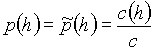where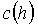is the count number of all occurrences of the edge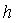, and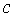is the count number of all occurrences of all edges in the training data sample. Similarly, we can estimate the bigram probabilities as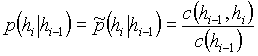where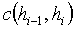is the count number of all occurrences of the edge pair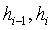(in the given order).

The trained probability distribution has one essential disadvantage: it is very sparse, : especially with the bigram model there are many edge pairs that have not occurred in the training data and so they get a probability of zero. But we should not simply forbid such pairs because once we might want to use the language model together with an additional source of information. If this additional info absolutely prefers one of those not-‘unseen’ pairs, and that pair has a probability slighter more higher than zero, it still can be selected. That is why we should avoid the zero probabilities. We have to smooth the distribution so that it does not contain any zero holes. With smoothing we will gain an additional advantage: we will be able to distinguish more softly subtly the edges that were seen in the training sample in a context different context than in from that of the test data.

We do that by adding the unigram probabilities to the bigram ones. So farAlbeit we have not yet seen the edge pair, it is still possible that we have seen the edge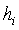alone. However, if we have not seen the edgeat all, it would gets again the zero probability. To avoid this case, we add also a constant, the uniform probabilitywhereis the size of the lexicon and thusis the count number of possible edges. Since the sum of three probabilities is not itself a probability (it can exceed 1), we have to weight each component by a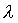from the interval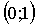; (the sum of all weights must be 1):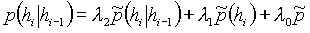In our experiments we use estimated values of weights. With bigram models we use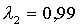,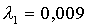, and. With the unigram model we use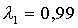, and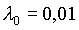.

The actual syntax syntactic analysis proper is performed in the second phase as we construct a the dependency tree. Here we use the trained and smoothed probability distribution to find the best, i.e. the most probable tree. We will construct the tree using the greedy algorithm. We start with a discrete graph, containing the root only. Then, in each step we add the edge that increases the tree probability at most. Note that not all remaining edges are allowed to be added in each step. O, but only those edges can be added that will not violate the correctness of the tree. Particularly, we are allowed to add those edges whose superior word has already been added to the tree, and the subordinated one has not. With the bigram models, we additionally have to reduce the allowable set to those edges that keep the given search order. By that we guarantee that the ”guard” probabilitywill be equal to 1. Note that the greedy algorithm does not guarantee thatis the highest possible value. Rather it is an approximation we use to reduce the computational complexity.

We have not specified yet how the training data look like that we have at our disposal look like. In particular, we must specify what we understand under a ”word”. It might be a lexical unit (lemma), a word form, a tag containing some morphological information, or any combination of the above. Since the available data resources are quite poor at the time (April 1997) we relinquish give up training any based on lexical information and concentrate ourselves to on the morphological tags. At the moment, we work with resources in which the tags are not disambiguated, it means so that most of the word forms have associated a set of tags associated with them. Exactly one of the elements of the set members is accurate correct in the given context but we do not know which one it is. That is why we have to deal with whole tag sets.

Both It is the matter of course that both the training and the parsing phases must be amended when training on disambiguated sets of tags. If there is an edge in the training sample whose superior governing node contains i and subordinate node contains j tags then we have to record allpossible combinations (tag edges). At once wWe have to remember that these combinations represent only one edge occurrence in the training data. In order to keep the relation to frequencies of the other edges, we distribute the occurrence among the particular tag combinations. E.g., if there is an edge with the superior node [solí, NFS7A|NFP2A|VPX3A] and the subordinated node [bílou, AFS41A|AFS71A] then that edge actually represents exactly one of the combinations NFS7A-AFS41A, NFS7A-AFS71A, NFP2A-AFS41A, NFP2A-AFS71A, VPX3A-AFS41A, and VPX3A-AFS71A. Instead of incrementing frequency of some edge by one, we raise the frequencies of each combination by. Then, during the parsing phase, we compute the probability of an event disjunction:The last equation member is equal to zero if no tag combination occurs in one edge more than once. It is true that in our data such a repetition is possible, but the reasons are not relevant for our task. So Therefore we are going to record every combination only once.

Similarly In the same view we have to deal with the context edges in bigram models. If the edgein scope contains the tag combinationsto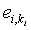and the its context edge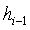in context contains the combinations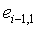to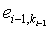then, in the training phase, we record each combination from the edge in scope gradually in contexts of all combinations from the context edge, each time with the frequency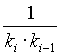. In the parsing phase, we have to remember how the probabilities are estimated by the frequencies. Because every edge frequency is actually a sum of frequencies of tag combinations, we get: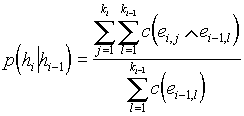We see that the ambiguity of morphological tags increases enormously the computational complexity, especially with the bigram distribution. Since certain words can have up to 27 possible tags, to estimate one probability value we may need about half a million accesses to the table to estimate one probability value!

9. Testing and Results
10. We have at our disposal sample data containing 1150 trees. We reserve 100 of them for testing purposes because the model cannot be tested on the same data it has been trained by. The rest 1050 trees (18015 words) form our training sample.

Table 1. Basic characteristics of particular models. T is the number of training trees. By ”param’s” we mean the number of different edges (or edge pairs respectively) that occurred in the training sample. The value is higher than the number of words with the unigram model, too, because some edges are formed labelled by sets of tag combinations. The entropy of a model is defined by the equation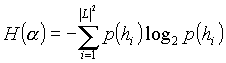for the unigram model and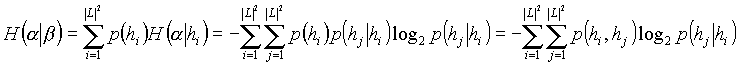for the bigram model. The perplexity is.

 Basic model characteristics words param’s entropy perplexity unigram, T=50 684 3127 9,43 689 unigram, T=250 3960 10 305 10,64 1599 unigram, T=1050 18 015 23 099 11,12 2227 bigram depth-first, T=50 684 78 026 2,51 6 bigram depth-first, T=250 3960 439 964 3,64 13 bigram depth-first, T=1050 18 015 1 901 858 bigram width-first, T=50 684 51 775 2,70 7 bigram width-first, T=250 3960 456 723 3,88 15 bigram width-first, T=1050 18 015 1 943 864

We have tested each model as followsin the following way: we took a tree from the test data, reconstructed the plain sentence (i.e. removed the tree structure) and made it the input of the parsing procedure. After parsing, we thus had got two trees, the old onefrom the data, and the new one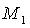, generated by the parser. Then we counted the wrong edges, i.e. the number of words that were subordinated to different nodes in both trees. We defined the success rate as the count number of all wrong edges in the test output, divided by the count number of all edges in the test data. Note that for this purpose we compared the word occurrences rather than the contents of the tag sets. Hence if two words had had the same tag sets, and the parser had swapped their positions in the tree, they both were counted as wrong edges.

Table 2. Again, T is the number of the training trees. Z is the number of the test trees.

 Success rate T = 50 Z = 5 T = 250 Z = 25 T = 1050 Z = 100 unigram 25 % 32 % 31 % bigram depth-first 29 % 22 % 30 % bigram width-first 23 % 23 % 27 %

Table 3. The cross entropy is defined as follows:for the unigram model and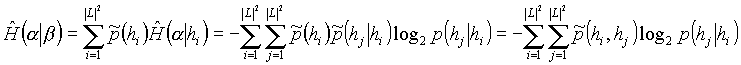for the bigram model. As before, the symbol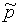denotes the relative frequency in the test data rather than probability. The higher the cross entropy is, the more edges of the test data are not known from the training data.

 Cross entropy T = 50 Z = 5 T = 250 Z = 25 T = 1050 Z = 100 unigram 21,68 14,84 12,60 bigram depth-first 21,98 10,81 bigram width-first 21,45 11,11

We see that the results are not very satisfying satisfactory. First surprise is the higher It is surprising that the error rate with bigrams is higher than that with unigrams. Most likely insufficient data resources cause this. However, it is also possible that the defined context has a minor influence in the parsing process. The disadvantage of limiting allowed edges by depth-first or width-first search order then comes to the foreground.

Our parser is only slighterly more successful than a procedure that assigns trees to sentences in a certain pre-defined way. After this procedure, each word depends on the preceding word in the sentence, only the first word and the final period depend on the root. We do not present any comparison here because in the experiments the error rate was measured in a different way in order to compare it to the work of Kiril Ribarov (1996). For details, please see the original dissertations of Daniel Zeman (1997) and Kiril Ribarov (1996).

An important question is whether the errors of our method are caused mostly by concessions made in the model definition or the by an inaccurate construction of the most probable tree. If the model (together with training data) is accurate, the original trees from the data will get higher probabilities than the wrong trees generated by the parser. We proofed have demonstrated experimentally that this is the weakness of our method, see the next table.

Table 4. The values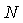, andare the count numbers of tested trees, and the count numbers of cases when the generated tree received a higher probability than the original one, respectively. We also define the difference of two trees as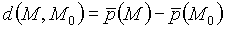where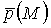is the probability of the tree M, normalised to the number of nodes as follows. Actually it is a geometrical average of edge probabilities.Actually it is a geometrical average of edge probabilities. Note that a positive difference indicates the generated tree being more probable, and a negative one indicates the opposite vice versa. In the tTable 4 we present average differences.

 Average probability difference between generated and original tree N N0 d(M,M0) unigram, T = 50 5 5 +6,0.10-3 unigram, T = 250 25 22 +2,9.10-3 unigram, T = 1050 100 89 +2,8.10-3 bigram depth-first, T = 50 5 5 +1,8.10-3 bigram width-first, T = 50 5 5 +2,0.10-3

Table 5. The last experiment shall will show the influence of the smoothing weights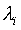. If it appeared significant, we could specify the weights by the EM algorithm instead of estimating them. We experimented with an unigram model, trained on 250 and tested on 25 sentences. Gradually we dropped the value ofand raised. We proofed have demonstrated that there is almost no influence on the error rate and, as the following figure shows, only a minor influence on the cross entropy.

11. Summary

With the data available in April 1997, none of the tested methods seems to be useful for parsing Czech. The Our observations made point to a weakness of the probability distribution rather than to a bad edge searching (the greedy algorithm). This does not mean, however, that the statistical approach should be completely thrown out over at all. We have made several simplifications and approximations, forced partly by the time and space computational complexity, partly just by the amount and quality of available data. Let us summarise now the some points of possible improvements, which may therefore become an object of further research. (The order of items does not necessarily correspond with to their importance necessarily.)

1. The model uses dependencies between morphological tags but ignores the relations between lexical units. For example, a comma in the sentence is marked by the same tag as any other interpunction punctuation mark, although it surely behaves other in a different way than, e.g., the final period full stop. An ”ideal” model should take into account a combination of lexical as well as morphological relations between the superior governing and subordinate dependent nodes, and, if possible, take at least an advantage of bigrams at least.
2. The fact that one node can have a few several variants of morphological tags is most likely a significant source of inaccuracy. For instance, the Czech word form ”V” may be not only a preposition at the beginning of the sentence begin but also a Rroman number symbol 5; however, in our model both derived edge groups receive the same weight. We can name list many defects of this type. Very often there are several combinations of number and case that can be assigned to a substantive or adjective word form. Exactly these attributes play a significant role in finding dependencies (the agreement between a name noun and its attribute or between the predicate and the subject). We believe that as soon as an unambiguous disambiguated morphological analysis (or tagging) will be is available, the precision will increases. At the same time the complexity of the computation should fall down.
3. In some cases we might use less and in other cases more specialised tags. For example, the difference between particular pronoun types should not have major influence on the syntax while their gender, number and case are quite important. On the other hand there is an essential difference between a comma and the final period full stop, but in our data the same code ZIP is assigned to both of them (see also point 1).
4. The smoothing weights were estimated. As shown in Tthe table 5, in under current circumstances this approximation makes no difference to the success rate of the model. However, certain changes of cross entropy in the same Ttable mark that with much more data we can expect an increasing influence of the smoothing weights. Then we could improve the result by training the weights with some variant of the EM algorithm.
5. We have not used all the information we had about the sentence. In particular, we should consider the word order, suppress the non-projective edges, and find a suitable way to use the distance between the superior and the subordinate nodes in the sentence.
6. In the work of Kiril Ribarov (1996), the tree is not constructed from an empty one. Instead, the sentence is deterministically structured to a start tree where each word depends on the preceding one, only the first word and the final period full stop depend on the root. Then the start tree is adjusted as long as there is an edge improving probability. It might be interesting to change our method this way,; however this probably will not improve anything before we construct a better probability distribution.
7. The training data contained errors — understand that is, errors according with respect to the rules a human uses to parse a sentence, because otherwise the ”truth” is defined just by the contents of the training sample. If these mistakes appear with the same frequency in the test data, their existence shall not hinder. (Examples: badly placed end of the sentence; sentence parsed to as a chain where each word depends on the preceding one.)
1. Acknowledgements
2. The research would not be possible without a corpus of manually annotated sentences (the training data). The (yet incomplete) corpus we used has been made available by the grant GAÈR No. 405/96/K214 and by the project of the Ministry of Education of the Czech Republic No. VS96151.

3. References
1. [Bahl et al. 1989]
Lalit Bahl, Peter Brown, Peter de Souza, Robert Mercer: A Tree-Based Statistical Language Model for Natural Language Speech Recognition. In: IEEE Transactions on Acoustics, Speech, and Signal Processing, vol. 37, No. 7, July 1989. Yorktown Heights 1989.
2. [Brown et al. 1993]
Peter Brown, Stephen della Pietra, Vincent della Pietra, Robert Mercer: The Mathematics of Machine Translation: Parameter Estimation. In: Computational Linguistics, 19 (2): 1993, pp. 263-312, 1993.
3. [Cover, Thomas 1991]
Thomas Cover, Joy Thomas: Elements of Information Theory. John Wiley & Sons, Inc., New York 1991.
4. [Dempster et al. 1976]
Arthur Dempster, N. M. Laird, Donald Rubin: Maximum Likelihood from Incomplete Data via the EM Algorithm. In: Journal of the Royal Statistical Society, Series B, Volume 39, London 1977, pp. 1-38. London 1977.
5. [Holan et al. 1995]
Kuboò, Martin Plátek: An Implementation of Syntactic Analysis of Czech. In: Fourth International Workshop on Parsing Technologies. Praha, 1995.
6. [Chomsky 1957]
Noam Chomsky: Syntactic Structures. Mouton & Co. Publishers, Den Haag 1957.
7. [Oliva 1989]
Karel Oliva: A Parser for Czech Implemented in Systems Q. Explicitní popis jazyka a automatické zpracování textu Explizite Beschreibung der Sprache und automatische Textverarbeitung XVI. Matematicko-fyzikální fakulta Univerzity Karlovy, Praha 1989.
8. [Petkeviè 1998]
Vladimír Petkeviè: Underlying Structure of Sentence Based on Dependency. Filozofická fakulta Univerzity Karlovy, Praha (in press).
9. [Ribarov 1996]
Kiril Ribarov:
Automatická tvorba gramatiky pøirozeného jazyka (dissertationdiploma thesis). Matematicko-fyzikální fakulta Univerzity Karlovy, Praha 1996.
10. [Sgall et al. 1969]